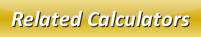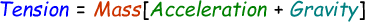# Tension Calculator

In physics, a tension is used to measure an objects capacity to withstand the stress and strain. For instance, when a rope is attached on an iron bar at one end and a is pulled by a vehicle on the other end of the rope.

We can calculate tension when we know the mass, acceleration and gravity. Here is the formula:Use our below online tension calculator to find the tension of a rope etc by inputting the required fields and then click calculate to find the answer.

 Mass: Acceleration: Gravity: Tension:

Latest Calculator Release

Average Acceleration Calculator

Average acceleration is the object's change in speed for a specific given time period. ...

Free Fall Calculator

When an object falls into the ground due to planet's own gravitational force is known a...

Torque Calculator

Torque is nothing but a rotational force. In other words, the amount of force applied t...

Average Force Calculator

Average force can be explained as the amount of force exerted by the body moving at giv...

Angular Displacement Calculator

Angular displacement is the angle at which an object moves on a circular path. It is de...